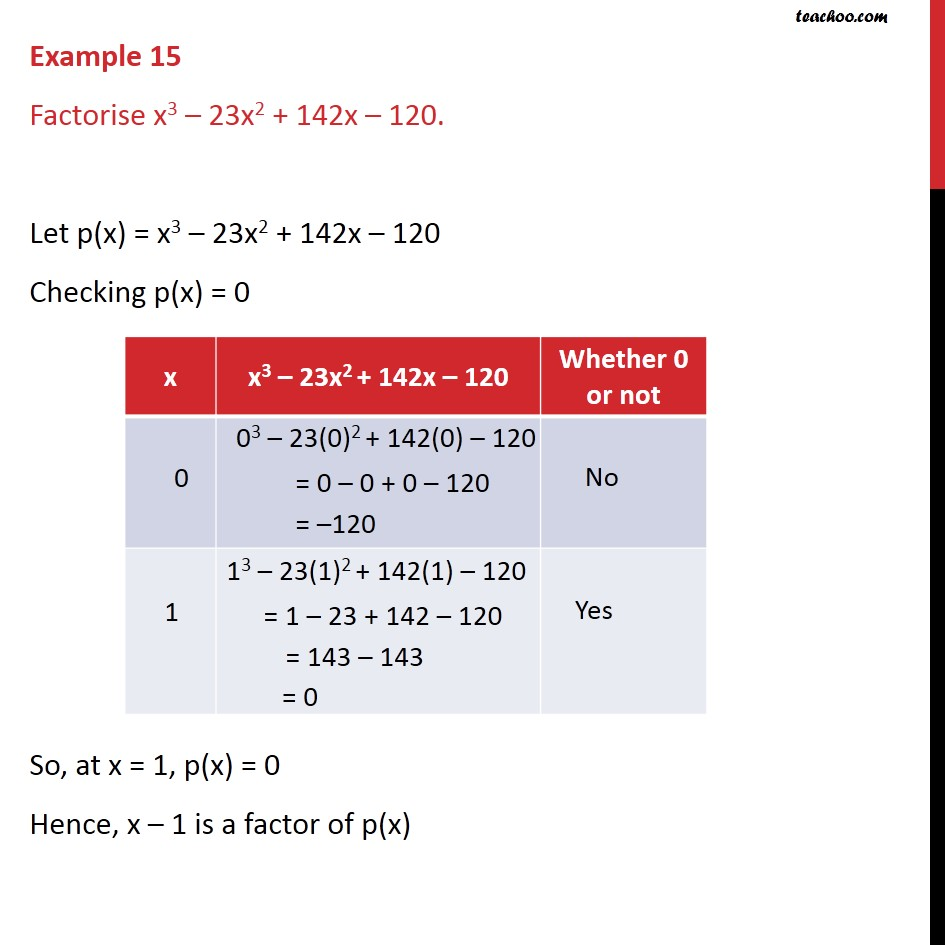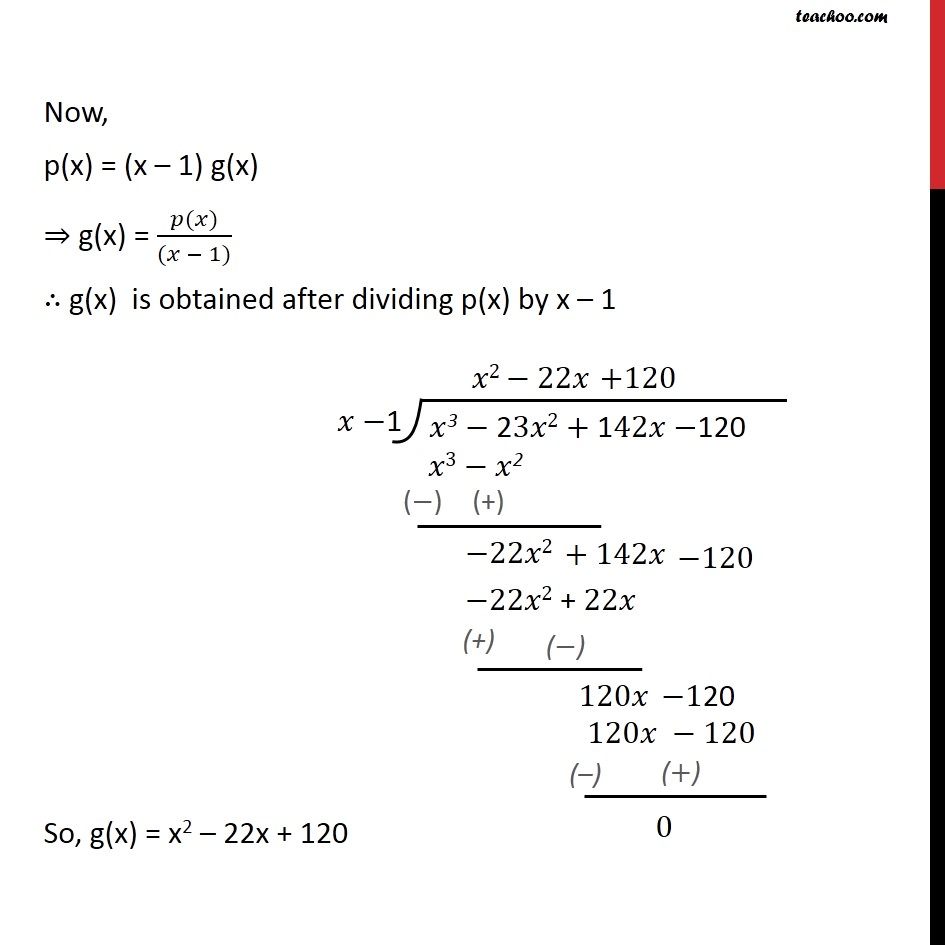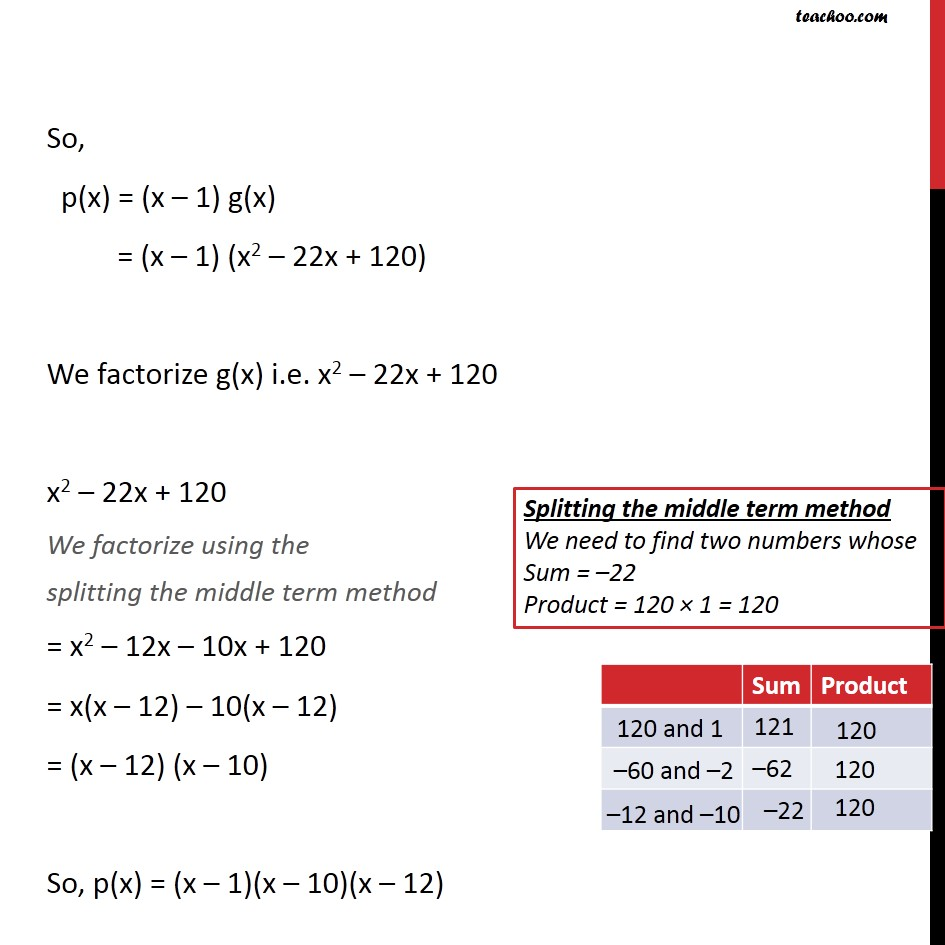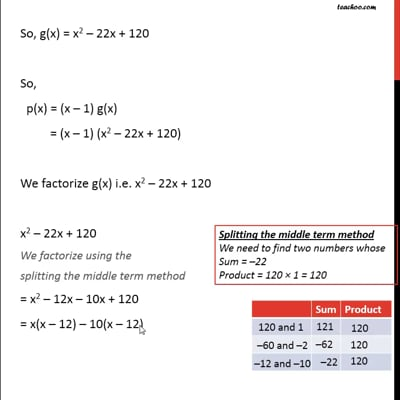Examples

Chapter 2 Class 9 Polynomials
Serial order wiseThis video is only available for Teachoo black users

Learn in your speed, with individual attention - Teachoo Maths 1-on-1 Class

### Transcript

Example 10 Factorise x3 23x2 + 142x 120. Let p(x) = x3 23x2 + 142x 120 Checking p(x) = 0 So, at x = 1, p(x) = 0 Hence, x 1 is a factor of p(x) Now, p(x) = (x 1) g(x) g(x) = ( ( ))/(( 1)) g(x) is obtained after dividing p(x) by x 1 So, g(x) = x2 22x + 120 So, p(x) = (x 1) g(x) = (x 1) (x2 22x + 120) We factorize g(x) i.e. x2 22x + 120 x2 22x + 120 We factorize using the splitting the middle term method = x2 12x 10x + 120 = x(x 12) 10(x 12) = (x 12) (x 10) So, p(x) = (x 1)(x 10)(x 12)